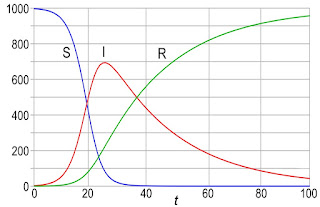Friday, November 13, 2020

The SIR Model of Epidemics

In Chapter 10 of Intermediate Physics for Medicine and Biology, Russ Hobbie and I discuss models described by nonlinear differential equations. We provide several examples in the text and homework problems, but one topic we never address is epidemics.

The archetype mathematical description of an epidemic is the SIR model. A population is divided into three categories, corresponding to three dynamic variables:

S: the number of susceptible people

I: the number of infected people

R: the number of recovered people.

Three differential equations govern the number of people in each category.

dS/dt = - (β/N) I S

dI/dt = (β/N) I Sγ I

dR/dt = γ I

where N is the total population, and β and γ are constants. Rather than analyze these equations myself, I’ll let you do it in a new homework problem.
Section 10.8

Problem 36 ½. The SIR model describes the dynamics of an epidemic.
(a) Add the three differential equations and determine how the total number of people (S + I + R) changes with time. Does this model include people who die from the disease?
(b) Write the equation governing the number of infected people as dI/dt = γI (r0 – 1). Find an expression for r0. Initially, when S = N, what does r0 reduce to? This value of r0 is known as the basic reproduction number. If r0 is less than what value will the number of infected people decay, preventing an epidemic?
(c) Suppose r0 is greater than one, so the number of infected people grows and the epidemic spreads. How low must the ratio S/N become for I to begin decreasing? Once this value of S/N is reached, the population is said to have herd immunity and the epidemic decays away.Results from a numerical simulation of the SIR model, using S(0) = 997, I(0) = 3, R(0) = 0, β = 0.4, and γ = 0.04. By Klaus-Dieter Keller, CC0, https://commons.wikimedia.org/w/index.php?curid=77633956

The SIR model provides insight into the COVID-19 pandemic. It’s a simple model, and many researchers have modified it to be more realistic. Yet, there is value in a toy model like SIR. It lets you to gain intuition about a dynamical system without being overwhelmed by complexity. I always encourage students to first master a toy model, and only then add additional detail.